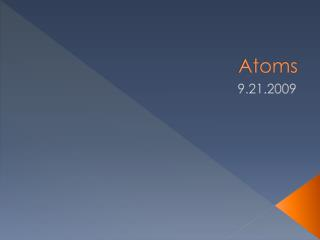DownloadDownload PresentationAtoms

Atoms

Download PresentationAtoms

- - - - - - - - - - - - - - - - - - - - - - - - - - - E N D - - - - - - - - - - - - - - - - - - - - - - - - - - -
Presentation Transcript

1. Atoms 9.21.2009

2. What is an Atom? • Just exactly what is an atom and why is it important? • An atom is the smallest particle into which an element can be divided and still keep its unique chemical properties • Atoms make up elements • Atoms are called the building blocks of matter

3. Just how BIG is an Atom? • Do you think you can see an atom with your eye? • Not at all • An atom is so small that it would take a stack of 50,000 aluminum atoms to equal the thickness of a sheet of aluminum foil

4. Did you know…. • An atom actually is made up of even smaller particles: • The nucleus • The nucleus is the central part of an atom • It consists of 2 parts: • 1. protons • 2. neutrons • Outside the nucleus • This is where the atom’s electrons are found

5. Atomic Structure

6. The Nucleus • The nucleus is made up of what 2 things? • 1. neutrons • Neutrons are ‘neutral’ • They have NO charge • 2. protons • Protons have a positive charge • Which particles in the diagram represent protons and which represent neutrons?

7. The Nucleus (Part 2) • The nucleus contains almost all of an atoms mass • Each proton has a mass of 1 atomic mass unit (abbreviated amu) • Each neutron also has a mass of 1 atomic mass unit (amu) • However, the nucleus has a very small volume

8. Electrons….The Outsiders • Electrons are typically found outside the nucleus within electron clouds • Electrons are high in energy and move around rapidly outside the nucleus • Electrons are negatively charged

9. It’s Lonely On the Outside • The mass of an electron is very small and considered to be insignificant • In a neutral atom (one with no overall charge) the number of electrons is equal to the number of protons

10. How Are Electrons Arranged? • Remember that electrons are found outside of an atoms nucleus • They move randomly within regions called electron clouds • Each electron cloud can only hold a certain number of electrons • The first electron cloud only holds 2 electrons • The second and third electron clouds hold 8 electrons each • You have to fill each cloud completely before the electrons can move to the next

11. The Big Picture • If all atoms are made out of the same things…..then how are they different from one another? • The key is the number of the particles present • The number of protons in an atom determines which element it is • The number of protons is the atomic number of that atom

12. Atomic Number • All atoms of an element will have the same atomic number • Examples: • Every hydrogen atom has only one proton • Every gold (Au) atom has 79 protons • Look at the periodic table and determine which of the numbers in the box corresponds to the atomic number. • Do you see a pattern?

13. Atomic Number

14. Atomic Number • You should have noticed that as the elements go from left to right, the atomic number increases by… • 1 • Ifthe atomic number is equal to the number of protons, what does that tell us? • That as we move from left to right, the number of protons in the nucleus also increases by 1

15. Atomic Number • Using your periodic table, find the element that has: • An atomic number of 41 • An atomic number of 22 • An atomic number of 16 • 31 protons • 18 protons

16. Atomic Mass • The atomic mass number is the sum of the number of protons plus neutrons in an atom • Why are electrons not included in an atoms atomic mass? • Remember that the mass of an electron is extremely small and insignificant

17. Atomic Mass • Looking at the picture below and determine which number is the atomic mass

18. Atomic Mass Examples • Atomic mass = ? • Neutrons + protons • How many neutrons does Yttrium have? • What is the atomic mass of Scandium? • If an atom has 7 protons and 7 neutrons, what is its atomic mass? What element is this?

19. Atomic Mass Examples • If Hafnium has 72 protons and an atomic mass of 178.5 amu’s, how many neutrons does it have? • Can an atom really have 106.5 neutrons? • NOOO!!!!!!! • The reason it is listed as being a decimal is because of a thing called an isotope

20. Iso WHO? • Isotopes are atoms that have the same number of protons but have DIFFERENT numbers of neutrons • So when you see a decimal in the atomic mass it is because the atomic mass of each isotope is used to determine an elements average atomic mass • Remember that it is the number of PROTONS that determines the elements